Tans in Squares

The following pictures show n tans with leg 1 packed inside the smallest known squares (of side s ).

 1.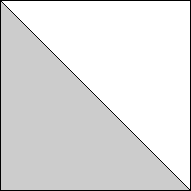2.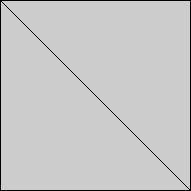3.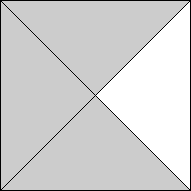s = 1Trivial. s = 1Trivial. s = √2 = 1.414+Trivial.

 4.5.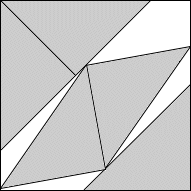6.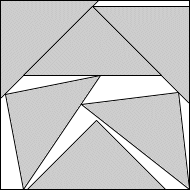s = √2 = 1.414+Trivial. s = 1.788+Found by Maurizio Morandiin August 2007. s = 1.937+Found by Maurizio Morandiin February 2008.

 7.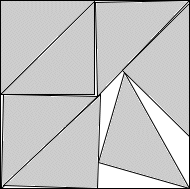8.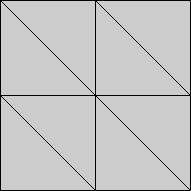9.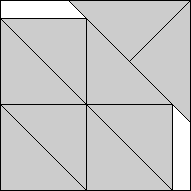s = 1.999+Found by Maurizio Morandiin August 2007. s = 2Trivial. s = 3/2 + 1/√2 = 2.207+Found by Erich Friedmanin March 2005.

 10.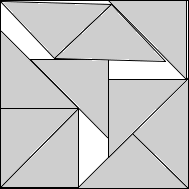11.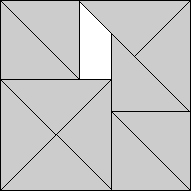12.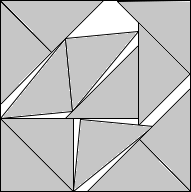s = 2.393+Found by Maurizio Morandiin January 2008. s = 1 + √2 = 2.414+Found by Maurizio Morandiin August 2007. s = 2.612+Found by Maurizio Morandiin January 2008.

 13.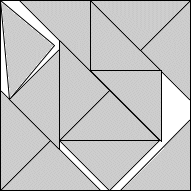14.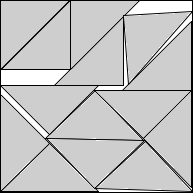15.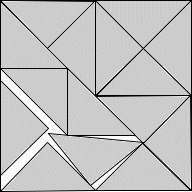s = 2.688+Found by Maurizio Morandiin March 2008. s = 2.767+Found by Maurizio Morandiin October 2007. s = 2.828+Found by Maurizio Morandiin August 2007.

 16.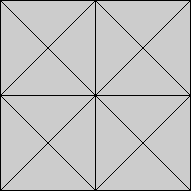17.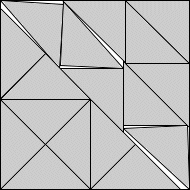18.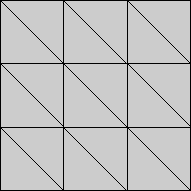s = 2√2 = 2.828+Trivial. s = 2.970+Found by David W. Cantrellin August 2007. s = 3Trivial.

 19.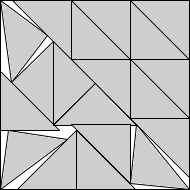20.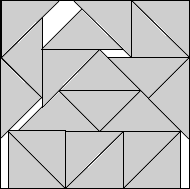21.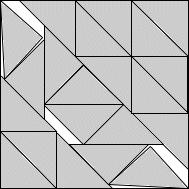s = 3.201+Found by Maurizio Morandiin February 2008. s = 2 + 9√2/10 = 3.272+Found by Maurizio Morandiin February 2008. s = 3.340+Found by Maurizio Morandiin March 2008.

 22.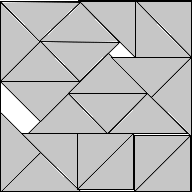23.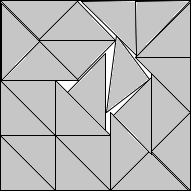24.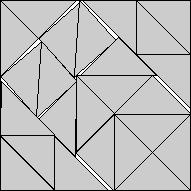s = 3.392+Found by Maurizio Morandiin December 2007. s = 3.464+Found by Maurizio Morandiin December 2007. s = 3.517+Found by David W. Cantrellin August 2007.